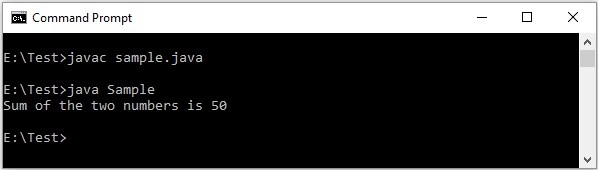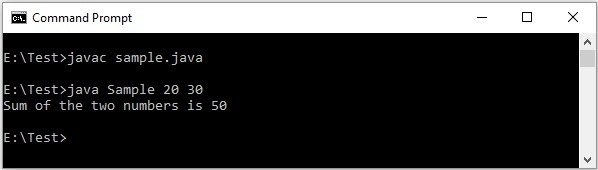# Explain Java command line arguments.

You can pass n number of arguments from the command line while executing the program, separating them with spaces.

java ClassName 10 20 30 . . . . .

The main method accepts/reads the values you have passed into an array of the type String.

public class
public static void main(String[] args){
}

These arguments are known as command line arguments.

## Using command line arguments

After writing a Java program we will compile it using the command javac and run it using the command javas. Consider the following code −

## Example

public class Sample{
public static void main(String[] args){
int a = 20;
int b = 30;
int c = a+b;
System.out.println("Sum of the two numbers is "+c);
}
}

We would compile and run it from command line as shown below −

## OutputHere, we are hard coding (fixing to a specific value) the values of a and b which is not recommendable. To handle this, you can accept parameters from user using classes from I/O package or Scanner.

Another way is to use command line arguments.

## Example

In the following example we are rewriting the above program, by accepting the (two integer) values from the command line. And, we are extracting these two from the String array in the main method and converting them to integer −

public class Sample {
public static void main(String[] args){
int a = Integer.parseInt(args);
int b = Integer.parseInt(args);
int c = a+b;
System.out.println("Sum of the two numbers is "+c);
}
}

## Output

You can compile and, run the program by passing the values at execution line through command prompt as shown below: